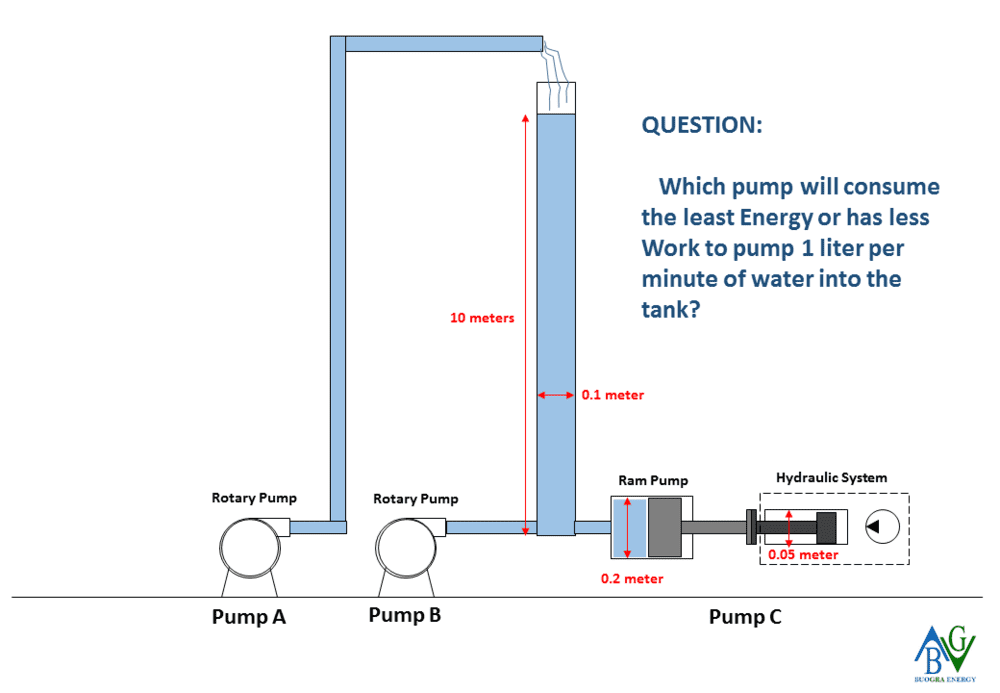# Water Tank Refilling via Hydraulic Water Pumping System

Don Bori
TL;DR Summary
Will hydraulic system lessen the input energy or work required in pumping water into the tank? If yes, why please?
Based on my understanding,

View attachment Top Tank Refilling
Disadvantage: High Head (Requires more distance, thus more Work since W = f x d)

Bottom Tank Refilling
Disadvantage: High Static Pressure (Requires more Force, thus more Work since W = f x d)

Therefore to address the disadvantage of Bottom Tank Refilling, will hydraulic system lessen the input energy or work required in pumping water into the tank? If yes, why please? Thank you in advance!

Don BoriStaff Emeritus
Clarify please. Are you asking about the total energy to fill the tank from empty to full?

Don Bori

The objective is only to top up the water tank to keep the water level.

Clarify please. Are you asking about the total energy to fill the tank from empty to full?

Mentor
You can solve this yourself.

Step 1: For each of the three cases, calculate the total pressure at the pump discharge. Total pressure is the static head plus line loss. You correctly recognize that Pump A has higher pressure than Pump B, but it's good practice to put numbers on it.

Step 2A: Calculate the efficiency of the hydraulic system. That includes the hydraulic pump efficiency plus line losses from the hydraulic pump to the hydraulic cylinder plus hydraulic cylinder friction. You will need to spend some time studying hydraulic catalogs.

Step 2B: Find the efficiency of Pumps A & B from their pump curves or data sheets.

Step 3: The input energy required is the work at the pump discharge divided by the pump efficiency and further divided by the motor efficiency.

•Don Bori
Don Bori
Appreciate this and will do calculations by considering this methods.

Will share with you the results.

You can solve this yourself.

Step 1: For each of the three cases, calculate the total pressure at the pump discharge. Total pressure is the static head plus line loss. You correctly recognize that Pump A has higher pressure than Pump B, but it's good practice to put numbers on it.

Step 2A: Calculate the efficiency of the hydraulic system. That includes the hydraulic pump efficiency plus line losses from the hydraulic pump to the hydraulic cylinder plus hydraulic cylinder friction. You will need to spend some time studying hydraulic catalogs.

Step 2B: Find the efficiency of Pumps A & B from their pump curves or data sheets.

Step 3: The input energy required is the work at the pump discharge divided by the pump efficiency and further divided by the motor efficiency.

I'm confused as to what the hydraulic system is even supposed to do in this arrangement. Filling from the bottom will definitely be more energy efficient than filling from the top, since top filling always requires pumping the water higher than the maximum fill level regardless of the water level in the tank. However, as far as I can tell, that hydraulic system isn't doing anything at all.

•Don Bori
Gold Member
The power out of the pump is the pressure (at the pump outlet) times the flow. The problem states that the flow is the same in the two cases. But, as you noted the pressures are not. So the answer is clear with regard to the hydraulic work at the pump outlet. If this isn't clear, consider the dimensions of pressure, flow, work...

•Don Bori
Don Bori
Let's consider the formula of Work = Force x Distance (elevation).

For Top Refilling it will be Less Force - High Distance, it is lesser Force because the discharge port is atmospheric pressure. And the static pressure is negligible if we use smaller diameter water pipes.

For Bottom Refilling it will the opposite, High Force - Less Distance due to High Fluid Static pressure.

So if the problem with Bottom Refilling is the High Force requirement to pump in the water, then I thought of supplementing it with Hydraulics because of its force-multiplier feature.

But I don't know if it will lesser the Work Done.

PS: Feel free to correct me, not a Physics expert here and just based my analysis to basic formulas.

Cheers!

I'm confused as to what the hydraulic system is even supposed to do in this arrangement. Filling from the bottom will definitely be more energy efficient than filling from the top, since top filling always requires pumping the water higher than the maximum fill level regardless of the water level in the tank. However, as far as I can tell, that hydraulic system isn't doing anything at all.

Don Bori
Appreciate your inputs, will try to do more basic calculations to share with you for your comments and suggestions.

Thanks!

The power out of the pump is the pressure (at the pump outlet) times the flow. The problem states that the flow is the same in the two cases. But, as you noted the pressures are not. So the answer is clear with regard to the hydraulic work at the pump outlet. If this isn't clear, consider the dimensions of pressure, flow, work...

Joe591
I believe pump B will be the most efficient.

Pump A lifts the water much too high and then it just drops to to the dam water level. All that extra work to lift the water higher than the dam level is wasted.

Pump C has way too many energy conversions. I'm assuming that the hydraulic pump is driven by a electrical motor. This means that the energy is converted from electrical to mechanical rotary motion. This is then converted to hydraulic power, this is then converted to linear motion in the ram, which is then converted back into hydraulic energy. Each energy conversion has a loss associated with it. This results in a very inefficient system.

•Don Bori
•